# confusionchart

Create confusion matrix chart for classification problem

## Syntax

``confusionchart(trueLabels,predictedLabels)``
``confusionchart(m)``
``confusionchart(m,classLabels)``
``confusionchart(parent,___)``
``confusionchart(___,Name,Value)``
``cm = confusionchart(___)``

## Description

example

````confusionchart(trueLabels,predictedLabels)` creates a confusion matrix chart from true labels `trueLabels` and predicted labels `predictedLabels` and returns a `ConfusionMatrixChart` object. The rows of the confusion matrix correspond to the true class and the columns correspond to the predicted class. Diagonal and off-diagonal cells correspond to correctly and incorrectly classified observations, respectively. Use `cm` to modify the confusion matrix chart after it is created. For a list of properties, see ConfusionMatrixChart Properties.```
````confusionchart(m)` creates a confusion matrix chart from the numeric confusion matrix `m`. Use this syntax if you already have a numeric confusion matrix in the workspace.```
````confusionchart(m,classLabels)` specifies class labels that appear along the x-axis and y-axis. Use this syntax if you already have a numeric confusion matrix and class labels in the workspace.```
````confusionchart(parent,___)` creates the confusion chart in the figure, panel, or tab specified by `parent`.```

example

````confusionchart(___,Name,Value)` specifies additional `ConfusionMatrixChart` properties using one or more name-value pair arguments. Specify the properties after all other input arguments. For a list of properties, see ConfusionMatrixChart Properties.```
````cm = confusionchart(___)` returns the `ConfusionMatrixChart` object. Use `cm` to modify properties of the chart after creating it. For a list of properties, see ConfusionMatrixChart Properties.```

## Examples

collapse all

```load fisheriris X = meas; Y = species;```

`X` is a numeric matrix that contains four petal measurements for 150 irises. `Y` is a cell array of character vectors that contains the corresponding iris species.

Train a k-nearest neighbor (KNN) classifier, where the number of nearest neighbors in the predictors (k) is 5. A good practice is to standardize numeric predictor data.

`Mdl = fitcknn(X,Y,'NumNeighbors',5,'Standardize',1);`

Predict the labels of the training data.

`predictedY = resubPredict(Mdl);`

Create a confusion matrix chart from the true labels `Y` and the predicted labels `predictedY`.

`cm = confusionchart(Y,predictedY);`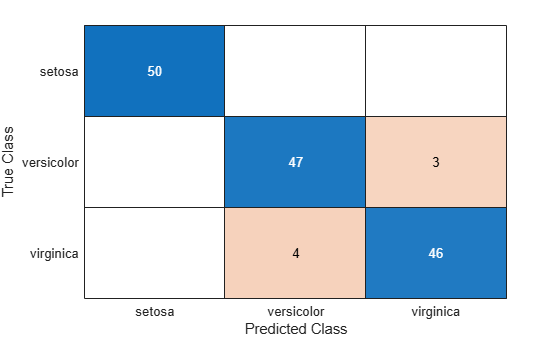The confusion matrix displays the total number of observations in each cell. The rows of the confusion matrix correspond to the true class, and the columns correspond to the predicted class. Diagonal and off-diagonal cells correspond to correctly and incorrectly classified observations, respectively.

By default, `confusionchart` sorts the classes into their natural order as defined by `sort`. In this example, the class labels are character vectors, so `confusionchart` sorts the classes alphabetically. Use `sortClasses` to sort the classes by a specified order or by the confusion matrix values.

The `NormalizedValues` property contains the values of the confusion matrix. Display these values using dot notation.

`cm.NormalizedValues`
```ans = 3×3 50 0 0 0 47 3 0 4 46 ```

Modify the appearance and behavior of the confusion matrix chart by changing property values. Add a title.

`cm.Title = 'Iris Flower Classification Using KNN';`

```cm.RowSummary = 'row-normalized'; cm.ColumnSummary = 'column-normalized';```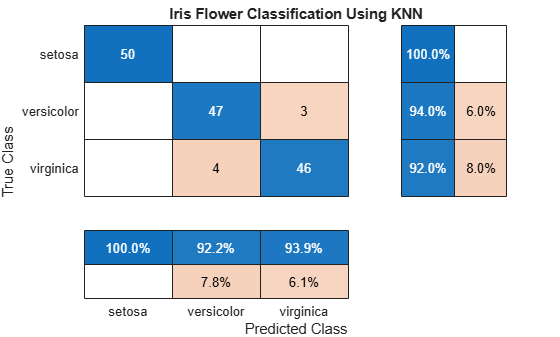A row-normalized row summary displays the percentages of correctly and incorrectly classified observations for each true class. A column-normalized column summary displays the percentages of correctly and incorrectly classified observations for each predicted class.

Create a confusion matrix chart and sort the classes of the chart according to the class-wise true positive rate (recall) or the class-wise positive predictive value (precision).

Load and inspect the `arrhythmia` data set.

```load arrhythmia isLabels = unique(Y); nLabels = numel(isLabels)```
```nLabels = 13 ```
`tabulate(categorical(Y))`
``` Value Count Percent 1 245 54.20% 2 44 9.73% 3 15 3.32% 4 15 3.32% 5 13 2.88% 6 25 5.53% 7 3 0.66% 8 2 0.44% 9 9 1.99% 10 50 11.06% 14 4 0.88% 15 5 1.11% 16 22 4.87% ```

The data contains 16 distinct labels that describe various degrees of arrhythmia, but the response (`Y`) includes only 13 distinct labels.

Train a classification tree and predict the resubstitution response of the tree.

```Mdl = fitctree(X,Y); predictedY = resubPredict(Mdl);```

Create a confusion matrix chart from the true labels `Y` and the predicted labels `predictedY`. Specify `'RowSummary'` as `'row-normalized'` to display the true positive rates and false positive rates in the row summary. Also, specify `'ColumnSummary'` as `'column-normalized'` to display the positive predictive values and false discovery rates in the column summary.

```fig = figure; cm = confusionchart(Y,predictedY,'RowSummary','row-normalized','ColumnSummary','column-normalized');```

Resize the container of the confusion chart so percentages appear in the row summary.

```fig_Position = fig.Position; fig_Position(3) = fig_Position(3)*1.5; fig.Position = fig_Position;```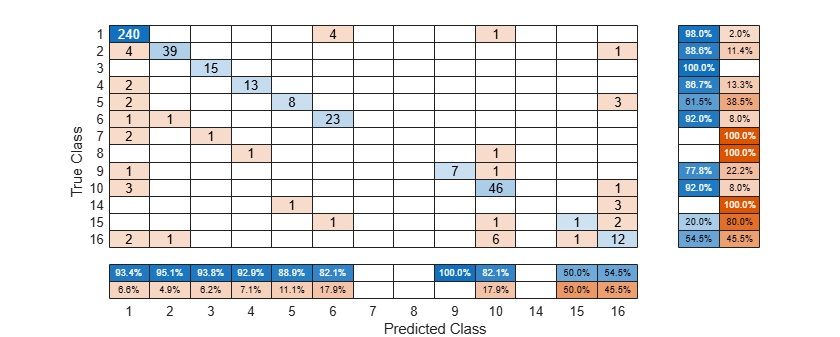To sort the confusion matrix according to the true positive rate, normalize the cell values across each row by setting the `Normalization` property to `'row-normalized'` and then use `sortClasses`. After sorting, reset the `Normalization` property back to `'absolute'` to display the total number of observations in each cell.

```cm.Normalization = 'row-normalized'; sortClasses(cm,'descending-diagonal') cm.Normalization = 'absolute'; ```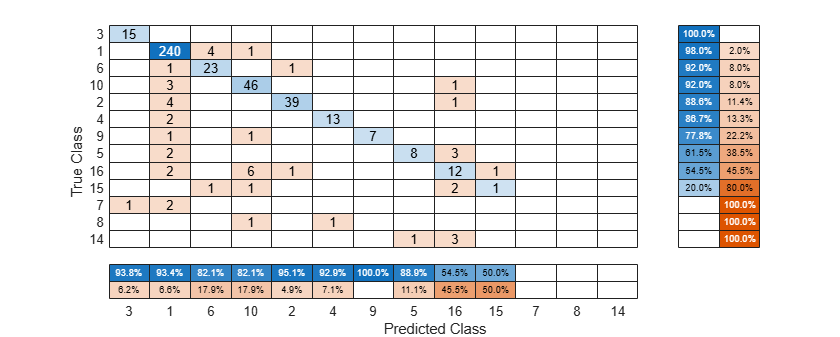To sort the confusion matrix according to the positive predictive value, normalize the cell values across each column by setting the `Normalization` property to `'column-normalized'` and then use `sortClasses`. After sorting, reset the `Normalization` property back to `'absolute'` to display the total number of observations in each cell.

```cm.Normalization = 'column-normalized'; sortClasses(cm,'descending-diagonal') cm.Normalization = 'absolute'; ```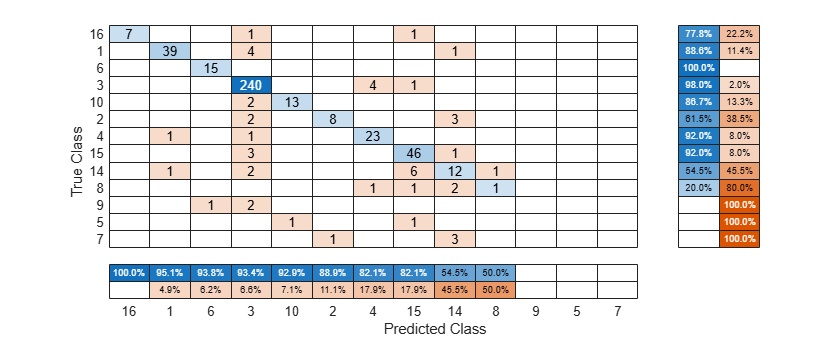Perform classification on a tall array of the Fisher iris data set. Compute a confusion matrix chart for the known and predicted tall labels by using the `confusionchart` function.

When you perform calculations on tall arrays, MATLAB® uses either a parallel pool (default if you have Parallel Computing Toolbox™) or the local MATLAB session. If you want to run the example using the local MATLAB session when you have Parallel Computing Toolbox, you can change the global execution environment by using the `mapreducer` function.

`load fisheriris`

Convert the in-memory arrays `meas` and `species` to tall arrays.

`tx = tall(meas);`
```Starting parallel pool (parpool) using the 'local' profile ... Connected to the parallel pool (number of workers: 4). ```
`ty = tall(species);`

Find the number of observations in the tall array.

`numObs = gather(length(ty)); % gather collects tall array into memory`

Set the seeds of the random number generators using `rng` and `tallrng` for reproducibility, and randomly select training samples. The results can vary depending on the number of workers and the execution environment for the tall arrays. For details, see Control Where Your Code Runs (MATLAB).

```rng('default') tallrng('default') numTrain = floor(numObs/2); [txTrain,trIdx] = datasample(tx,numTrain,'Replace',false); tyTrain = ty(trIdx); ```

Fit a decision tree classifier model on the training samples.

`mdl = fitctree(txTrain,tyTrain); `
```Evaluating tall expression using the Parallel Pool 'local': - Pass 1 of 2: Completed in 4.2 sec - Pass 2 of 2: Completed in 2.4 sec Evaluation completed in 11 sec Evaluating tall expression using the Parallel Pool 'local': - Pass 1 of 4: Completed in 1.7 sec - Pass 2 of 4: Completed in 2 sec - Pass 3 of 4: Completed in 3.5 sec - Pass 4 of 4: Completed in 2.9 sec Evaluation completed in 13 sec Evaluating tall expression using the Parallel Pool 'local': - Pass 1 of 4: Completed in 0.78 sec - Pass 2 of 4: Completed in 1.8 sec - Pass 3 of 4: Completed in 2.9 sec - Pass 4 of 4: Completed in 2.1 sec Evaluation completed in 9.6 sec Evaluating tall expression using the Parallel Pool 'local': - Pass 1 of 4: Completed in 1.2 sec - Pass 2 of 4: Completed in 1.9 sec - Pass 3 of 4: Completed in 3.3 sec - Pass 4 of 4: Completed in 2.4 sec Evaluation completed in 12 sec Evaluating tall expression using the Parallel Pool 'local': - Pass 1 of 4: Completed in 1.1 sec - Pass 2 of 4: Completed in 1.7 sec - Pass 3 of 4: Completed in 3.1 sec - Pass 4 of 4: Completed in 2.9 sec Evaluation completed in 12 sec ```

Predict labels for the test samples by using the trained model.

```txTest = tx(~trIdx,:); label = predict(mdl,txTest);```

Create the confusion matrix chart for the resulting classification.

```tyTest = ty(~trIdx); cm = confusionchart(tyTest,label)```
```Evaluating tall expression using the Parallel Pool 'local': - Pass 1 of 1: Completed in 0.67 sec Evaluation completed in 1.3 sec Evaluating tall expression using the Parallel Pool 'local': - Pass 1 of 1: Completed in 2 sec Evaluation completed in 3.1 sec ```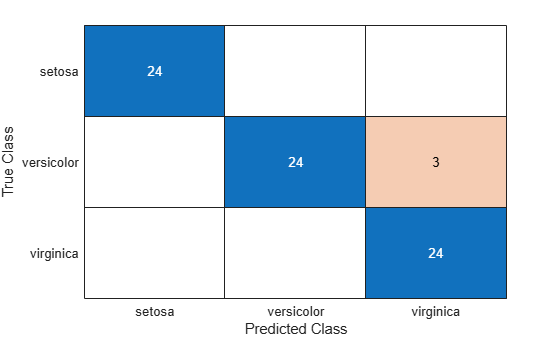```cm = ConfusionMatrixChart with properties: NormalizedValues: [3x3 double] ClassLabels: {3x1 cell} Show all properties ```

The confusion matrix chart shows that three measurements in the versicolor class are misclassified. All the measurements belonging to setosa and virginica are classified correctly.

## Input Arguments

collapse all

True labels of classification problem, specified as a categorical vector, numeric vector, string vector, character array, cell array of character vectors, or logical vector. If `trueLabels` is a vector, then each element corresponds to one observation. If `trueLabels` is a character array, then it must be two-dimensional with each row corresponding to the label of one observation.

Predicted labels of classification problem, specified as a categorical vector, numeric vector, string vector, character array, cell array of character vectors, or logical vector. If `predictedLabels` is a vector, then each element corresponds to one observation. If `predictedLabels` is a character array, then it must be two-dimensional with each row corresponding to the label of one observation.

Confusion matrix, specified as a matrix. `m` must be square and its elements must be positive integers. The element `m(i,j)` is the number of times an observation of the `i`th true class was predicted to be of the `j`th class. Each colored cell of the confusion matrix chart corresponds to one element of the confusion matrix `m`.

Class labels of the confusion matrix chart, specified as a categorical vector, numeric vector, string vector, character array, cell array of character vectors, or logical vector. If `classLabels` is a vector, then it must have the same number of elements as the confusion matrix has rows and columns. If `classLabels` is a character array, then it must be two-dimensional with each row corresponding to the label of one class.

Parent container in which to plot, specified as a `Figure`, `Panel`, or `Tab` object.

### Name-Value Pair Arguments

Specify optional comma-separated pairs of `Name,Value` arguments. `Name` is the argument name and `Value` is the corresponding value. `Name` must appear inside quotes. You can specify several name and value pair arguments in any order as `Name1,Value1,...,NameN,ValueN`.

Example: ```cm = confusionchart(trueLabels,predictedLabels,'Title','My Title Text','ColumnSummary','column-normalized')```

### Note

The properties listed here are only a subset. For a complete list, see ConfusionMatrixChart Properties.

Title of the confusion matrix chart, specified as a character vector or string scalar.

Example: ```cm = confusionchart(__,'Title','My Title Text')```

Example: `cm.Title = 'My Title Text'`

Column summary of the confusion matrix chart, specified as one of the following:

OptionDescription
`'off'`Do not display a column summary.
`'absolute'`Display the total number of correctly and incorrectly classified observations for each predicted class.
`'column-normalized'`Display the number of correctly and incorrectly classified observations for each predicted class as percentages of the number of observations of the corresponding predicted class. The percentages of correctly classified observations can be thought of as class-wise precisions (or positive predictive values).
`'total-normalized'`Display the number of correctly and incorrectly classified observations for each predicted class as percentages of the total number of observations.

Example: `cm = confusionchart(__,'ColumnSummary','column-normalized')`

Example: `cm.ColumnSummary = 'column-normalized'`

Row summary of the confusion matrix chart, specified as one of the following:

OptionDescription
`'off'`Do not display a row summary.
`'absolute'`Display the total number of correctly and incorrectly classified observations for each true class.
`'row-normalized'`Display the number of correctly and incorrectly classified observations for each true class as percentages of the number of observations of the corresponding true class. The percentages of correctly classified observations can be thought of as class-wise recalls (or true positive rates).
`'total-normalized'`Display the number of correctly and incorrectly classified observations for each true class as percentages of the total number of observations.

Example: `cm = confusionchart(__,'RowSummary','row-normalized')`

Example: `cm.RowSummary = 'row-normalized'`

Normalization of cell values, specified as one of the following:

OptionDescription
`'absolute'`Display the total number of observations in each cell.
`'column-normalized'`Normalize each cell value by the number of observations that has the same predicted class.
`'row-normalized'`Normalize each cell value by the number of observations that has the same true class.
`'total-normalized'`Normalize each cell value by the total number of observations.

Modifying the normalization of cell values also affects the colors of the cells.

Example: `cm = confusionchart(__,'Normalization','total-normalized')`

Example: `cm.Normalization = 'total-normalized'`

## Limitations

• MATLAB® code generation is not supported for `ConfusionMatrixChart` objects.

﻿